# Dixon test to detect outliers in Excel tutorial

2017-10-20

This tutorial will help you run a Dixon test to detect outliers and interpret the results in Excel using XLSTAT.
Not sure this is the statistical test you are looking for? Check out this guide.

## Dataset for testing outliers with Dixon test

An Excel sheet with both the data and the results can be downloaded by clicking here.

The data have been obtained from a normal distribution with mean 0 and variance 3. One outlier has been added. We wish to test if there is one outlier in the sample.

## Goal of this tutorial

We would like to detect one or two outliers from a sample using Dixon test.

## Setting up a Dixon test to detect an outlier

To start the Dixontest go to the menu Testing outliers / Dixon test.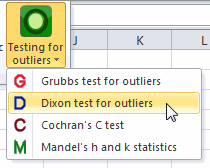In the General tab, select the data and the automatic option.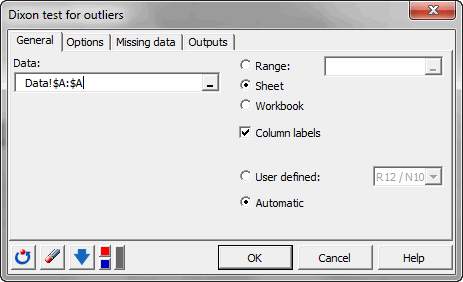As an alternative hypothesis choose the two-sided option. The default significance level is left as is: 5%. The p-value is obtained with a Monte Carlo simulation approach. We choose to use 1000000 simulations.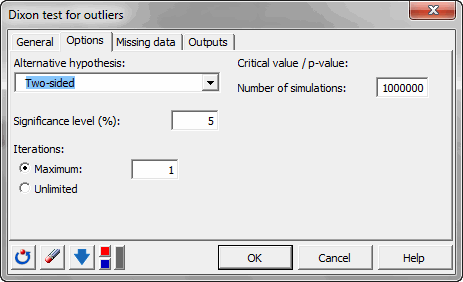## Results of a test for detecting an outlier

The result is that the p-value for this test is smaller than 0.0001. That means that the null hypothesis should be rejected.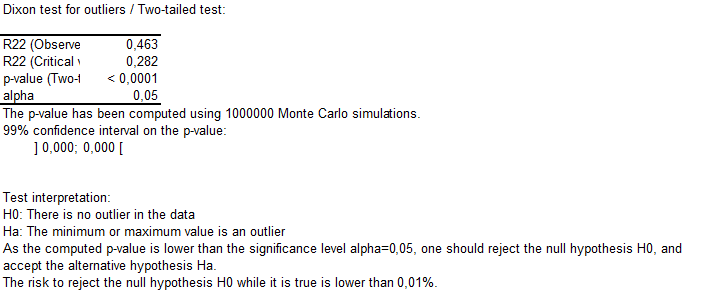In the following table, the detected outliers are given.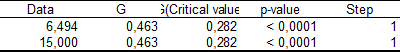You can also find the Z score to detect outliers in the output of this test.

#### Contact our technical support team: support@xlstat.com

https://cdn.desk.com/
false
desk